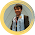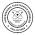### QUIZ #2 - OHM's LAW

ECT Movement - March 16, 2012

Quiz #2

For a given source of  120 volts and having  resistance of 20ᾩ  which is connected in
series  with the  shunt resistances of 30ᾩ and 60ᾩ respectively in the circuit, find?

1)the  total flow of electrons passing  through the conductor of  a circuit
a.1A
b. 2A
c.3A
d. 4A

2)  how much flow of electrons in 30 ohms resistor
a.2A
b.3A
c.4A
d. 5A

3) total power dissipated  by the resistances
a.360 Watts
b.361 Watts
c. 362 Watts
d.363 Watts

4) If a current of 3 A is divided in the circuit which is  consist of two parallel resistors with values of 8 ohms and 4 ohms respectively , the current flowing through the 4 Ohm resistor is …
a.  3 A
b.  2 A
c.  1.5 A
d. 1 A

5) In  a circuit into which a current I is flowing and consists  of  6 ohms, 4 ohms and 10 ohms connected in series to  each other . Which ammeter shows the highest reading when you connect  in the circuit to each resistors respectively?
a.  A1
b.  A2
c.  A3
d. All three ammeters give the same reading

6) In a circuit containing an ohmic resistor, a voltmeter and an ammeter. If the reading on the ammeter A increases the reading on voltmeter V …
a. increases in the same ratio.
b. increases, but not in the same ratio
c. remains unchanged
d. decreases in the same ratio

7)In the circuit  B1, B2 and B3 are identical light bulbs. The internal resistance of the battery can be ignored. Which statement is true regarding the relative brightness of the bulbs if one bulb connected in series with  two parallel bulbs  ?
a. The three bulbs glow with the same brightness.
b. B2 and B3 glow with the same brightness but brighter than B1.
c. B2 and B3 glow with the same brightness but less brightly than B1.
d. B1 glows brighter than B2 while B2 in turn glows brighter than B3.

8) A 9 V battery is composed of six 1,5 V cells, which are connected in series. Each cell has an internal resistance of 0,2 Ω. What is the highest current that can be obtained from such a battery?
7.5 A
1.5 A
1.2 A
0.3 A

9) In  AC circuit which consists of RL load with values of R= 70Ω, XL= 60Ω respectively  and RC load with R= 40Ω, Xc= 25Ω that are connected in parallel  with a source of 230 VAC  of 60  hertz. Find the combined impedance?
a. Z= 37-j5.4Ω
b. Z= 37+j5.6Ω
c. Z= 37-j5.9Ω
d Z= 37+j 5.9Ω

Given that Z1= 200 − 40j Ω and Z2= 60 + 130j Ω connected in parallel with 12 volts AC source.Find the following?

10) total impedance
a. 87.2- j60.6Ω
b.87.4- j60.1Ω
c.87.2+ j60.6Ω
d.87.2-j60.6Ω

11) the phase angle
a.35
b36
c.37
d.38

12) total line current
a.0.113 polar angle of -34.82 A
b. 0.113 polar angle of 34.82 A
c. 0.114 polar angle of 34.83 A
d. 0.114 polar angle of -34.82A

A 100 Ω resistor, a 0.0200 H inductor and a 1.20 µF capacitor are connected in parallel with a circuit made up of a 110 Ω resistor in series with a 2.40 µF capacitor. A supply of 150 V, 60 Hz is connected to the circuit.

13) Calculate the total current taken from the supply
a. 204 mA
b. 203 mA
c. 204 mA
d. 205 mA

14) its phase angle
a. 86 degress
b. 85 degress
c. 84 degress
d. 87 degrees

15)Given that the current in a given circuit is 3.90 - 6.04j mA and the impedance is 5.16 + 1.14j kΩ, find the magnitude of the voltage
a.36 Volts
b.37 Volts
c. 38 Volts
d. 39 Volts

16) A resistor, an inductor and a capacitor are connected in series across an ac voltage source. A voltmeter measures 12.0 V, 15.5 V and 10.5 V respectively, when placed across each element separately. What is the magnitude of the voltage of the source?
a. 12.2 Volts
b. 13 Volts
c. 14 Volts
d. 15 Volts

Given in AC circuit that have 4 ohms resistance and 3 ohms reactive inductance.

17)What is the magnitude of impedance
a.6
b.5
c. 7
d. 8

18)polar angle
a.34 degrees
b. 36.87 degrees
c. 36.57 degress
d. 37.4 degress

19) What is the average power dissipation in an RLC series circuit with R = 10Ω, L = 0.1 H, C = 10 μF when driven at resonance by a 100 V-rms source?
a. 100 W
b. 500 W
c. 1000 W
d. 2 kW

20) A high-voltage powerline operates at 500 000 V-rms and carries an rms current of 500 A. If the resistance of the cable is 0.050Ω/km, what is the resistive power loss in 200 km of the powerline?
a. 250 kW
b. 500 kW
c. 1 Megawatt
d. 2.5 Megawatts

21) A 10-μF capacitor is plugged into a 110 V-rms 60-Hz voltage source, with an
ammeter in series. What is the rms value of the current through the capacitor?
a. 0.202 A (rms)
b. 0.415 A (rms)
c. 0.626 A (rms)
d. 0.838 A (rms)

22) A 0.5-H inductor is connected into a 110 V-rms 60-Hz voltage source, with an ammeter in series. What is the rms value of the current through the inductor?
a. 0.189 A (rms)
b. 0.292 A (rms)
c. 0.584 A (rms)
d. 1.19 A (rms)

23) The inductance of a tuning circuit of an AM radio is 4 mH. Find the capacitance of the circuit required for reception at 1200 kHz.
a. 2.1 pF
b. 4.4 pF
c. 21.2 pF
d. 43.4 pF

In a given AC circuit which consist 240 Volts AC 60 cycle per second and has Load connected across it. The wattmeter and ammeter connected also which gives reading  values of 1.5kW and 9.615 A(rms) respectively. Find the

24)Reacitve power of the circuit
a. 1.745 kVAR
b. 1.754 kVAR
c. 1.760 kVAR
d. 1.770 KVAR

25) required value of capacitor to connect in order to correct the poor power factor of a circuit  which improve greater system efficiency
80.761 uF
81.675 uF
82.761 uF
83.876 uF

source: Engr. Rito Banan

1.pwede po ba malaman ang mga sagot ng questions. Thank You po

2.u can directly contact Engr. Rito Banan thru email & Facebook.

3.1.